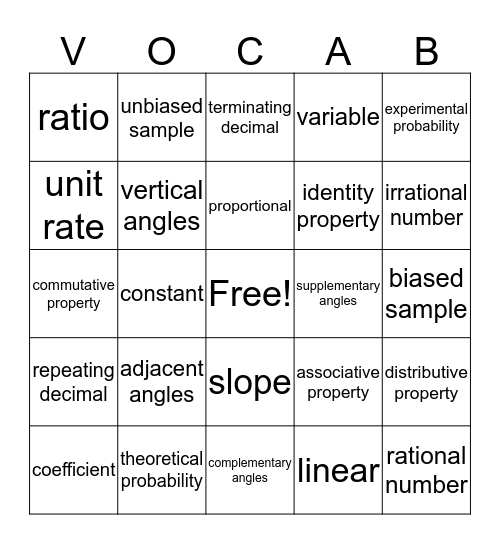Math 7 BingoThis bingo card has a free space and 26 words: slope, linear, proportional, theoretical probability, experimental probability, ratio, unit rate, unbiased sample, biased sample, associative property, identity property, commutative property, distributive property, coefficient, constant, integer, variable, repeating decimal, terminating decimal, rational number, irrational number, complementary angles, supplementary angles, adjacent angles, vertical angles and congruent.

Play Online